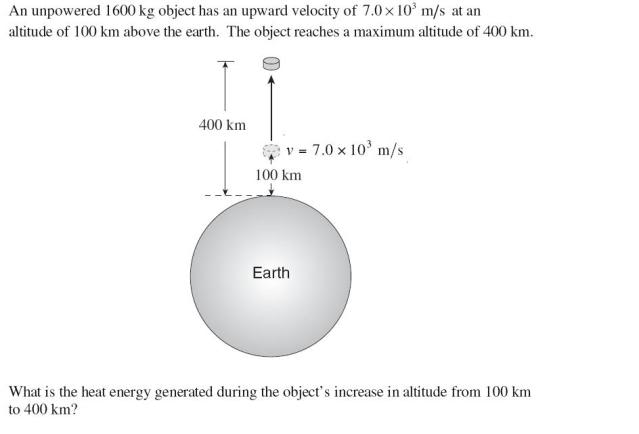# Heat energy

how do you do heat energy questions? is it the energy lost?

An unpowered 1600 kg object has an upward velocity of 7.0 x 10^3 m s at an
altitude of 100 km above the earth. The object reaches a maximum altitude of 400 km.
Earth
100 km
400 km
v = 7.0 × 10^3 m s

What is the heat energy generated during the object’s increase in altitude from 100 km
to 400 km?
A. 3.3 x 10^10 J
B. 3.4 x 10^10 J
C. 3.5 x 10^10 J
D. 5.5 x 10^10 J

this is from a sample exam, my teacher didnt teach us any of it.

I used EP= Gm1m2/(r^2) for each position, and ek = .5 mv^2 and subtracted the two and got a totally wrong answer.... Please help me.heres a pic....

also would solving this be similar to how you would find the heat lost from a resistor in a circuit because I had a question like that and I couldnt solve it.

I read that the change in potential is = work/energy and since velocity is constant EK is constant.
but when I use the formula for ep I dont get the right answer.

rock.freak667
Homework Helper
Well for one,

$$E_P= \frac{GM}{r}$$

at the max height, kinetic energy=0.

so at the start is velocity the 7E3 or is that the average velocity

Ive tried finding Etotal at the start and at the end and subtract one from the other but I always get the wrong answer.

Andrew Mason
Homework Helper
so at the start is velocity the 7E3 or is that the average velocity

Ive tried finding Etotal at the start and at the end and subtract one from the other but I always get the wrong answer.
Write out the equation for the total energy of the object as a function of R if there was no loss of energy. Determine what its KE would be at 400 km altitude. Compare that to the actual energy at 400 km (KE = 0). Where did that energy go?

AM

Start

Etotal=1/2mv^2 + (Gm1m2)/r
1/2•1600•(7E3)^2
(6.67E-11•1600•5.98E24)/(100•1000)
Etotal=6.36E12

Final

Etotal=EP+EK
6.36E12=(6.67E-11•5.98E24•1600)/(400•1000)+EK
EK=4.82E12 ?

whats wrong?

LowlyPion
Homework Helper
Start

Etotal=1/2mv^2 + (Gm1m2)/r
1/2•1600•(7E3)^2
(6.67E-11•1600•5.98E24)/(100•1000)
Etotal=6.36E12

Final
Etotal=EP+EK
6.36E12=(6.67E-11•5.98E24•1600)/(400•1000)+EK
EK=4.82E12 ?

whats wrong?

You need to use the radius from the center of Earth

I keep getting 4.36E10 though.

LowlyPion
Homework Helper

I keep getting 4.36E10 though.

I approach it as what should the velocity be.

1/2*Vi2 - μ/ri = 1/2*Vf2 - μ/rf

Using the Standard parameter for earth of 400,000 (which is in km3)

1/2*(49) - 400,000/6370 = 1/2*Vf2 - 400,000/ 6770

That yields 1/2*Vf2 = 24.5 - 62.79 + 59.084 = 20.79

Since that's already 1/2*Vf2 then multiply by the mass giving 33,270. But those units are in km2/sec2 so you need to properly convert to get J I'd think.

Andrew Mason
Homework Helper
Start

Etotal=1/2mv^2 + (Gm1m2)/r
1/2•1600•(7E3)^2
(6.67E-11•1600•5.98E24)/(100•1000)
Etotal=6.36E12

Final

Etotal=EP+EK
6.36E12=(6.67E-11•5.98E24•1600)/(400•1000)+EK
EK=4.82E12 ?

whats wrong?
You are using R to be 100 and 400 km. This is not correct. What is R? And where is it measured from?

AM

You are using R to be 100 and 400 km. This is not correct. What is R? And where is it measured from?

AM

Ya from the center of the earth, somebody else mentioned it but I still get the wrong answer, its getting closer though....but still wrong.

Andrew Mason